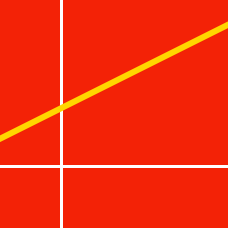Geometry

# Collinearity

If three points $A=(k,k+2),$ $B=(0,k-6)$ and $C=(k-4,k)$ all lie on the same line, what is the value of $k?$

The $x$- and $y$-intercepts of a line have the same value. If the line passes through $P=(7,-6)$ and $Q=(-6,k),$ what is the value of $k?$

Consider the two points $(-1, -1)$ and $(3, 7).$ If a circle passes through these two points, then which of the following cannot also be a point on the circle?

What is the sum of all the values of $k$ that make three points $A=(1,k),$ $B=(k,1)$ and $C=(10,10)$ lie on the same line?

Consider the line passing through two points $P=(1, 10)$ and $Q=(-1, 3)$. If the line also passes through point $R=(17, a)$, what is the value of $a$?

×